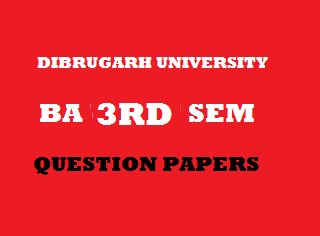## Friday, January 04, 20192014
(November)
EDUCATION
(Major)
Course: 302
(Educational Measurement and Evaluation)
Full Marks: 80
Pass Marks: 32
Time: 3 hours
The figures in the margin indicate full marks for the questions

1. Choose the right answers from the alternatives given below: 1x8=8
1. The percentage of case that lie betweenandof the normal probability curve is
1. 66.28
2. 66.26
3. 68.26
4. 68.28
1. If the IQ of a child is 130 and his chronological age is 10, then what will be his mental age?
1. 12 years.
2. 12.5 years.
3. 13 years.
4. 13.5 years.
1. If the mean and median of an achievement test are 45.55 and 44.75, then what will be its mode?
1. 43.15
2. 43.25
3. 43.35
4. 43.45
1. Which one of the following is not a characteristic of CCE?
1. Specifying learning outcomes of the learners.
2. Collection of evidences about pupils’ achievement.
3. Analysis and interpretation of collected data.
4. Limited emphasis on feedback.
1. Which one of the following statements is not true regarding standardized achievement test?
1. It is prepared on the basis of a blueprint.
2. Items are selected on the basis of indices of difficulty and discrimination.
3. It is not necessary to calculate reliability of the test.
4. It is accompanied by norm tables.
1. The number of pictures in Murray’s Thematic Apperception Test (TAT) is
1. 15
2. 20
3. 25
4. 30
1. The most dependable measure of variability is
1. Range.
2. Standard deviation.
3. Average deviation.
4. Quartile deviation.
1. When the scores are massed at the higher end of normal probability curve, then it is called as
1. Positive skewness.
2. Negative skewness.
3. Leptokurtic.
4. Platykurtic.
2. What is meant by achievement test? Discuss the steps need to be followed in construction of standardized achievement test. 3+8=11
Or
What is meant by the term ‘reliability’? Discuss the factors that affect reliability. 3+8=11
3. What is meant by the term ‘measurement’? Illustrate with suitable examples the functions of measurement. 3+8=11
Or
Name the different scales of measurement and describe the salient features of any one of them. Illustrate the relationship between measurement and evaluation with suitable examples. 2+4+5=11
4. Discuss the importance of Rorschach test and Thematic Apperception Test in the assessment of personality.   5+5=10
Or
Discuss with suitable examples the general aptitude test battery. 10
5. Calculate mean, median, and mode from the following frequency distribution table given below: 4+4+2=10
 Class intervals Frequency 10-20 20-30 30-40 40-50 50-60 60-70 70-80 3 5 7 10 8 4 3 N = 40

Or
Calculate the mean deviation and standard deviation from the frequency distribution table given above: 5+5=10
6. In a sample of 800 cases, the mean of a certain test is 60 and standard deviation is 10.00. Assuming normality of the distribution, find the number of individuals who scored between 30 and 50. [Percentage of scores which lie between mean andand between mean and]
Or
The following are the marks obtained by 10 students in two school subjects:
 Students Subject – I Subject – II A B C D E F G H I J 45 33 56 70 35 56 33 80 70 49 38 56 57 77 58 77 56 77 56 37

Compute the coefficient of correlation by rank-difference method and interpret the result.   5+1=6
7. Draw a histogram from the frequency distribution given in Question No. 5. 3
8. Write short notes on the following: 4x5=20
1. Scope of measurement in education.
2. Meaning of tools in evaluation.
3. Verbal and nonverbal tests.
4. Scope of educational statistics.
5. Properties of normal probability curve.

***